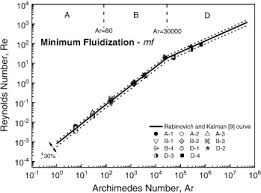## How to Calculate and Solve for Reynold’s Number at Minimum Fluidization | FluidizationThe image above represents reynold’s number.

To compute for reynold’s number, four essential parameters are needed and these parameters are Fluidization at Minimum Velocity (umf), Diameter of Bed (d), Density (ρ) and Viscosity (μ).

The formula for calculating reynold’s number:

Re’mf = umf/μ

Where:

Re’mf = Reynold’s Number at Minimum Fluidization
umf = Fluidization at Minimum Velocity
d = Diameter of Bed
ρ = Density
μ = Viscosity

Let’s solve an example;
Find the reynold’s number at minimum fluidization when the fluidization at minimum is 14, the diameter of bed is 8, the density is 12 and the viscosity is 2.

This implies that;

umf = Fluidization at Minimum Velocity = 14
d = Diameter of Bed = 8
ρ = Density = 12
μ = Viscosity = 2

Re’mf = umf/μ
Re’mf = (14)(8)(12)/2
Re’mf = (1344)/2
Re’mf = 672

Therefore, the reynold’s number at minimum fluidization is 672.

Calculating the Fluidization at Minimum Velocity when the Reynold’s Number at Minimum Fluidization, the Diameter of Bed, the Density and the Viscosity is Given.

umf = Re’mf x μ /

Where;

umf = Fluidization at Minimum Velocity
Re’mf = Reynold’s Number at Minimum Fluidization
d = Diameter of Bed
ρ = Density
μ = Viscosity

Let’s solve an example;
Find the fluidization at minimum velocity when the reynold’s number at minimum fluidization is 12, the diameter of bed is 4, the density is 8 and the viscosity is 3.

This implies that;

Re’mf = Reynold’s Number at Minimum Fluidization = 12
d = Diameter of Bed = 4
ρ = Density = 8
μ = Viscosity = 3

umf = Re’mf x μ /
umf = 12 x 3 / (4)(8)
umf = 36 / 32
umf = 1.125

Therefore, the fluidization at minimum velocity is 1.125.